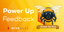# Outline of a brief introduction to statistics and learning

in #abundancetribe3 months ago

What is learning and what is the relation or connection to mundane statistics and experience?

One short answer could be the following.

(a) Probability is properly considered a functor from a category to a comma category (Gromov), rather than merely an additive normalized measure (Bayes, Kolmogorov), that best conserves objects while maximizing the simplification achieved.

Comment. In other words, think of a transformation rule T that takes a complicated graph with many connections or arrows with a simpler graph, with fewer connections and arrows. However, there are further weights added to each edge, not merely a generator of objects or operation as previously, and there is a decision rule D on these weights (Wald) that tells how to deterministically follow a path when it forks. Previously in the complicated graph this was open ended such that any path that generated grouplike the desired object A starting with a given object B was allowed, equivalent to ordinary deduction (Hertz, Gentzen). The pair then (T,D) helps classify if stable when data grown and connective in a logically additive sense if two simplified graphs can be connected and the same (T,D) followed over the joint graph after connected. There is a loss function, such that taking a step costs something but getting A starting with B gains something, and maximizing the score per divergence from reproducing on a blackboard the objects of the complicated graph is the procedure. This is totally constructive --- computable.

(b) This is the best estimate of the next "small" stage of a continuous path given only the numerous data of the path so far and its environment and having access to no other reasoning, the "true" estimate of how the path will continue for a little bit further even if it does something different because conditions changed in a hidden way (Harrod, Savage). In other words, "ideal" interpolation and approximation and extrapolation (Winston). The simplification process per se often does coincide with ordinary deduction in many cases however, deduction as construed using computation rules to grow a tree and prune it when duly noting and joining branches as vectors (sentences, sequents) to which computable inference rules are applied (Hertz, Gentzen, Girard, Negri, Von Plato, etc), giving a transition and inference from one logical sentence to another, and this is done entirely with vectors. Doing this several times (layers) provides some abstractive logical inference capability statistically. The interpolation, approximation, and extrapolation often will do the equivalent of some sequent calculus with sequents abstracted from the data and deduced according to the data interpolated and extrapolated a little bit, especially when repeating the simplification procedure on an layer that is already simplified, learning/training being searching for weights, equivalently simplification rules, until the simplification rule used stabilizes while the (tacit) decision rule is basically held constant and data available is updated and grows in amount.

Example. Some of the reasoning and natural language capabilities of many machine learning system 1970--2020 so far as these system have any such capabilities.

Comment. In other words, ordinary statistics using probabilities derived from vast data (Price already had asked in the preface to the paper by Bayes had asked how vast should be vast?) can for large data coincide with abstraction and deductive inference on sentences if repeating the procedure over many layers recursively, coinciding in some cases with comprehension (Kintsch) of natural language and many other things so long as they can be represented by vectors.

(c) What do you gain by moving beyond vast data plus simple neurons to more complex neurons that can do some reasoning each, agent-like? GOFAI-like?

One short answer could be the following.

Among the things gained ultimately is the ability to learn in terms of the decision rule too, it no longer has to be fixed. This helps with actual understanding. Less susceptible to procedural adversarial examples.

Frequency resolves to paths that exist.

We can recover the entire frequentist apparatus from this model.

The purpose of probability is to infer given global data including that about a single event to reconstruct what would happen in a single trial that went into that global data, for example, e.g., estimate what would happen to one event in a single trial knowing the frequencies of all events in many trials, infer from global to local (Bayes). Hence not per se connected to uncertainty or certainty, future or past, like the frequentist interpretation works also for deterministic series and where everything known, no difference between situation where individual data already known and where not known.

Probability is closely connected to a loss function, to some value (Bayes, Legendre, Savage).

Sort:

Upvoted by GITPLAIT!You distributed more than 42000 upvotes. Your next target is to reach 43000 upvotes.
If you no longer want to receive notifications, reply to this comment with the word `STOP`HiveBuzz supports meetups of the Hive UK CommunityFeedback from the September 1st Hive Power Up Day